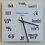# 17 or 19?

Does anyone have a good and short proof for $17^{19} > 19^{17}$ without using any help from calculators or log tables?Note by Muzaffar Ahmed
6 years, 10 months ago

This discussion board is a place to discuss our Daily Challenges and the math and science related to those challenges. Explanations are more than just a solution — they should explain the steps and thinking strategies that you used to obtain the solution. Comments should further the discussion of math and science.

When posting on Brilliant:

• Use the emojis to react to an explanation, whether you're congratulating a job well done , or just really confused .
• Ask specific questions about the challenge or the steps in somebody's explanation. Well-posed questions can add a lot to the discussion, but posting "I don't understand!" doesn't help anyone.
• Try to contribute something new to the discussion, whether it is an extension, generalization or other idea related to the challenge.
• Stay on topic — we're all here to learn more about math and science, not to hear about your favorite get-rich-quick scheme or current world events.

MarkdownAppears as
*italics* or _italics_ italics
**bold** or __bold__ bold
- bulleted- list
• bulleted
• list
1. numbered2. list
1. numbered
2. list
Note: you must add a full line of space before and after lists for them to show up correctly
paragraph 1paragraph 2

paragraph 1

paragraph 2

[example link](https://brilliant.org)example link
> This is a quote
This is a quote
    # I indented these lines
# 4 spaces, and now they show
# up as a code block.

print "hello world"
# I indented these lines
# 4 spaces, and now they show
# up as a code block.

print "hello world"
MathAppears as
Remember to wrap math in $$ ... $$ or $ ... $ to ensure proper formatting.
2 \times 3 $2 \times 3$
2^{34} $2^{34}$
a_{i-1} $a_{i-1}$
\frac{2}{3} $\frac{2}{3}$
\sqrt{2} $\sqrt{2}$
\sum_{i=1}^3 $\sum_{i=1}^3$
\sin \theta $\sin \theta$
\boxed{123} $\boxed{123}$

## Comments

Sort by:

Top Newest

Absolutly cz 17 is smaller than 19

- 6 years, 10 months ago

Log in to reply

No

- 6 years, 10 months ago

Log in to reply

Show that for $n \geq 3$, we have $n^{ \frac 1 {n+1}}$ is a decreasing sequence and that $n^{ \frac 1 {n-1}}$ is an increasing sequence. The usual approach would be calculus, but you can also use the binomial theorem.

Hence, we have $17^{19}>18^{18}>19^{17}$

Staff - 6 years, 10 months ago

Log in to reply

(This doesn't work out ... Yet)

A non calculus, non logarithm, non tedious expansion / calculation approach.

Step 1: Show using the binomial theorem that for $n\geq 3$, we have $n^{n+1}>(n+1)^n$. ( Do you see why you need n greater than 3?).

Step 2: compare $17^{18}> 18^{17}$ and $18^{19} > 19^{18}$.

Staff - 6 years, 10 months ago

Log in to reply

Ah, yes, a decent solution.. Thanks Bro @Calvin Lin :)

- 6 years, 10 months ago

Log in to reply

17^{17+2} or (17+2)^{17} Which is greater...?

- 6 years, 10 months ago

Log in to reply

Consider the function $f(x) = \displaystyle x^{\frac{1}{x}}$. If you test it's derivatives, you'll find it assumes it's maximum at $x=e$. So $f$ is larger for that number which is closer to $e$ as $f' <0$ for $x>e$. Particularly here, $e< 17 < 19$, so $17^{\frac{1}{17}} > 19^{\frac{1}{19}} \Rightarrow 17^{19}>19^{17}$. Sorry bro, calculus is the best option for these problems. You may use a non calculus number theoretic approach but that will be long. Explicitly, you need to do some calculation.

- 6 years, 10 months ago

Log in to reply

That's what we learnt in our school. For our exams... Nice example

- 6 years, 10 months ago

Log in to reply

\begin{aligned} \log 17^{19} &= 19\log 17 \\ &=\left(17\frac{19}{17}\right)\left(\log 19 \frac{\log 17}{\log 19}\right) \\ &= \underbrace{\left(\frac{\frac{\log 17}{17}}{\frac{\log 19}{19}}\right)}_{>1 \text{ because }\frac{\log x}{x}\text{ is strictly decreasing for }x>e }17\log 19 \\ \end{aligned} Therefore \begin{aligned} 19 \log 17 &> 17\log 19 \\ 17^{19} &> 19^{17} \end{aligned}

Staff - 6 years, 10 months ago

Log in to reply

Yeah I thought about that.. But.. Is there no other way we can prove that without using the rule of $\frac{log x}{x}$ is decreasing for $x > e$ ?

- 6 years, 10 months ago

Log in to reply

×

Problem Loading...

Note Loading...

Set Loading...